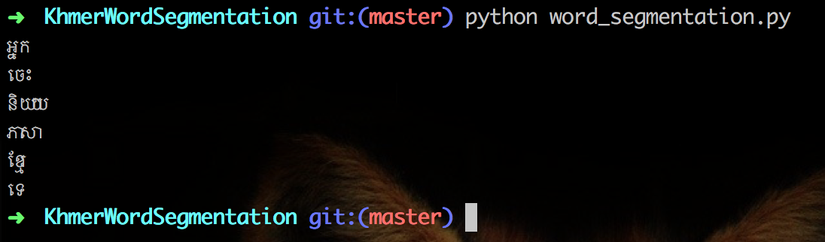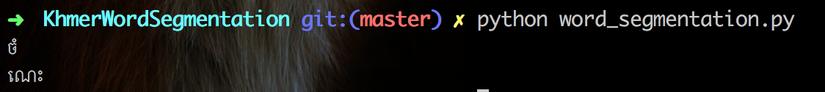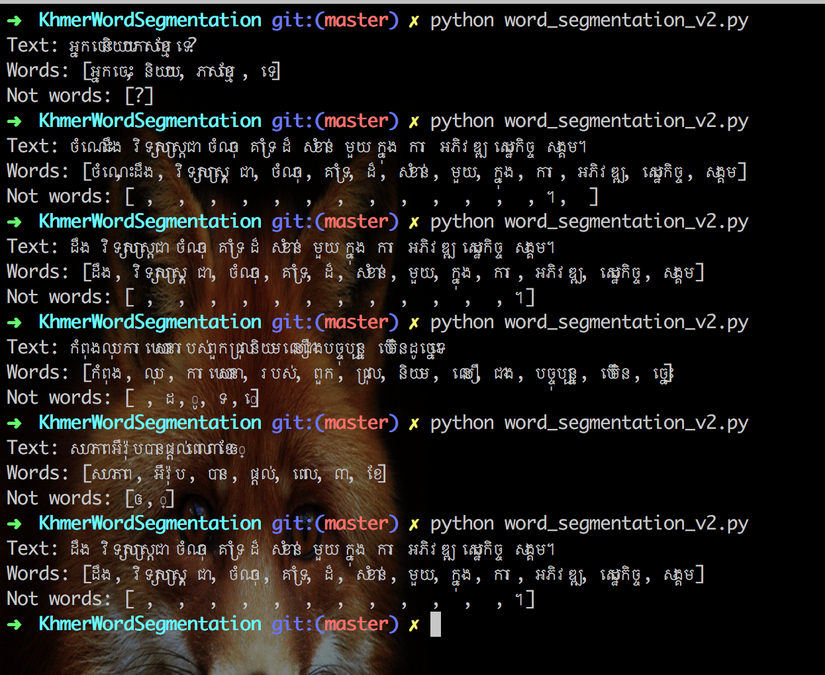+5

# NLP: Khmer Word Segmentation(part I)

In my last article, I had implemented a code to break Khmer sentences into an array of words. It works well when the sentences container words where we already train from start to the end of sentences. However, it cannot break a word from the sentence when there is an extracted character in front of it.

When all words are trained words. Sample: "អ្នកចេះនិយាយភាសាខ្មែរទេ?"When some words/character doesn't have in our trained model. Sample: "ចំណេះ​ដឹង​វិទ្យាសាស្ត្រ​ជា​ចំណុច​គាំទ្រ​ដ៏​សំខាន់​មួយ​ក្នុង​ការ​អភិវឌ្ឍ​សេដ្ឋកិច្ច​សង្គម។ "### Refactoring

Therefore, in this article we are going to implement a new version(v2) of word segmentation. I had been trying to research from many sources but I found not document for helping to solve this issuse. Luckily, when I discause this issue with Mr. Visal who is a Khmerload CTO, he gave me many awesome suggestions and most importantly his repo where he had created to solve his issue which similar to our article. So now, let implement our `word_segmentation_v2.py`.

First we create a class and its init function.

``````#!/usr/bin/python
# -*- coding: utf-8 -*-
#import trie model
import trie
from codecs import open, decode

class WordSegmentation:
# init Trie class
def __init__(self, text):
self.text = text.decode('utf-8')
self.model = trie.Trie()
# load trained model from file
# init list of found words
self.result = []
# init list of found words
self.leftover = []
# init start index
self.startIndex = 0
``````

Then let's create parse function where it loops and checks characters in given sentence. By first, it will check character for applying a correct parase function.

``````class WordSegmentation:
...
def parse(self):
while(self.startIndex < len(self.text)):
ch = self.text[self.startIndex]
ch = ch.encode('utf-8')
word = ''

if self.isNumber(ch):
word = self.parseNumber(self.startIndex).encode('utf-8')
elif self.isEnglish(ch):
word = self.parseEnglish(self.startIndex).encode('utf-8')
else:
word = self.parseTrie(self.startIndex)

length = len(word.decode('utf-8'))
if length == 0:
self.leftover.append(ch.decode('utf-8'))
self.startIndex += 1
continue

if self.model.searchWord(word) or self.isNumber(ch) or self.isEnglish(ch):
self.result.append(word.decode('utf-8'))
else:
self.leftover.append(word.decode('utf-8'))

self.startIndex += length
``````

If a character is number or English where it needs to use `parseNumber` or `parseEnglish` logic.

``````class WordSegmentation:
...
def isNumber(self, ch):
# number letter
return ch in "0123456789០១២៣៤៥៦៧៨៩"

def parseNumber(self, index):
result = ""
while (index < len(self.text)):
ch = self.text[index]
ch = ch.encode('utf-8')
if self.isNumber(ch):
result += self.text[index]
index += 1
else:
return result

return result
def isEnglish(self, ch):
return ch in "abcdefghijklmnopqrstuvwxyzABCDEFGHIJKLMNOPQRSTUVWXYZ"

def parseEnglish(self, index):
result = ""
while (index < len(self.text)):
ch = self.text[index]
ch = ch.encode('utf-8')
if (self.isEnglish(ch) or self.isNumber(ch)):
result += ch;
index += 1
else:
return result
return result
``````

Else we will check the character with our train model to confirm it's a word or not.

``````  def parseTrie(self, index):
word = ''
foundWord = ''

while (index < len(self.text)):
ch = self.text[index]
ch = ch.encode('utf-8')
word += ch
if self.model.searchWordPrefix(word):
if self.model.searchWord(word):
foundWord = word
elif self.model.searchWord(word):
return word
else:
return foundWord;

index += 1

return ""
``````

Let's put it all together:

``````#!/usr/bin/python
# -*- coding: utf-8 -*-

import trie
from codecs import open, decode

class WordSegmentation:
# init Trie class
def __init__(self, text):
self.text = text.decode('utf-8')
self.model = trie.Trie()
self.result = []
self.leftover = []
self.startIndex = 0

def isNumber(self, ch):
# number letter
return ch in "0123456789០១២៣៤៥៦៧៨៩"

def parseNumber(self, index):
result = ""
while (index < len(self.text)):
ch = self.text[index]
ch = ch.encode('utf-8')
if self.isNumber(ch):
result += self.text[index]
index += 1
else:
return result

return result
def isEnglish(self, ch):
return ch in "abcdefghijklmnopqrstuvwxyzABCDEFGHIJKLMNOPQRSTUVWXYZ"

def parseEnglish(self, index):
result = ""
while (index < len(self.text)):
ch = self.text[index]
ch = ch.encode('utf-8')
if (self.isEnglish(ch) or self.isNumber(ch)):
result += ch;
index += 1
else:
return result
return result

def parseTrie(self, index):
word = ''
foundWord = ''

while (index < len(self.text)):
ch = self.text[index]
ch = ch.encode('utf-8')
word += ch
if self.model.searchWordPrefix(word):
if self.model.searchWord(word):
foundWord = word
elif self.model.searchWord(word):
return word
else:
return foundWord;

index += 1

return ""

def parse(self):
while(self.startIndex < len(self.text)):
ch = self.text[self.startIndex]
ch = ch.encode('utf-8')
word = ''

if self.isNumber(ch):
word = self.parseNumber(self.startIndex).encode('utf-8')
elif self.isEnglish(ch):
word = self.parseEnglish(self.startIndex).encode('utf-8')
else:
word = self.parseTrie(self.startIndex)

length = len(word.decode('utf-8'))
if length == 0:
self.leftover.append(ch.decode('utf-8'))
self.startIndex += 1
continue

if self.model.searchWord(word) or self.isNumber(ch) or self.isEnglish(ch):
self.result.append(word.decode('utf-8'))
else:
self.leftover.append(word.decode('utf-8'))

self.startIndex += length

# # write to file
# with open('result/segment_word.txt', "w", encoding="utf-8") as f:
#   for word in self.result:
#     f.write(word + "\n")
# with open('result/segment_not_word.txt', "w", encoding="utf-8") as f:
#   for word in self.leftover:
#     f.write(word + "\n")

def show(self):
print('Text: ' + self.text)
print('Words: [' + ', '.join(self.result) + ']')
print('Not words: [' + ', '.join(self.leftover) + ']')

# kh_text = "អ្នកចេះនិយាយភាសាខ្មែរទេ?"
# kh_text = "ចំណេះ​ដឹង​វិទ្យាសាស្ត្រ​ជា​ចំណុច​គាំទ្រ​ដ៏​សំខាន់​មួយ​ក្នុង​ការ​អភិវឌ្ឍ​សេដ្ឋកិច្ច​សង្គម។ "
kh_text = "ដឹង​វិទ្យាសាស្ត្រ​ជា​ចំណុច​គាំទ្រ​ដ៏​សំខាន់​មួយ​ក្នុង​ការ​អភិវឌ្ឍ​សេដ្ឋកិច្ច​សង្គម។"
# kh_text = "កំពុងលុបការឃោសនារបស់ពួកជ្រុលនិយមលឿនជាងបច្ចុប្បន្ន បើមិនដូច្នេះទេ"
# kh_text = "សហភាពអឺរ៉ុបបានផ្ដល់ពេល៣ខែឲ្"

word_segment = WordSegmentation(kh_text)
word_segment.parse()
word_segment.show()
``````

Now let run and test our refactor with the text below:

• "អ្នកចេះនិយាយភាសាខ្មែរទេ?"
• "ចំណេះ​ដឹង​វិទ្យាសាស្ត្រ​ជា​ចំណុច​គាំទ្រ​ដ៏​សំខាន់​មួយ​ក្នុង​ការ​អភិវឌ្ឍ​សេដ្ឋកិច្ច​សង្គម។ "
• "ដឹង​វិទ្យាសាស្ត្រ​ជា​ចំណុច​គាំទ្រ​ដ៏​សំខាន់​មួយ​ក្នុង​ការ​អភិវឌ្ឍ​សេដ្ឋកិច្ច​សង្គម។"
• "កំពុងលុបការឃោសនារបស់ពួកជ្រុលនិយមលឿនជាងបច្ចុប្បន្ន បើមិនដូច្នេះទេ"
• "សហភាពអឺរ៉ុបបានផ្ដល់ពេល៣ខែឲ្"Yes, it works. As you can see now it break words from sentence very well. Very Awesome.

### Conclussion

Finally, our model can break words from sentences much better than before. However, it needs to train and test more in order to fit and improve our code even more. So that, we will be able to use it for our next lesson.